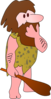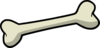Click here for the Problem Extension Worksheet version of the Problems of the Week.
Click here for an MS Word version of the Problems of the Week.
Click here for the Canadian Problem Extension Worksheet version of the Problems of the Week.
Click here for a Canadian MS Word version of the Problems of the Week.Lower Elementary:
Question: A woolly mammoth weighs 6 tons. A ton is 2,000 pounds. If a caveman weighs 200 pounds, then how many pounds heavier is the woolly mammoth than the caveman?
Answer: 11,800 pounds
Solution: The woolly mammoth weighs 2,000 + 2,000 + 2,000 + 2,000 + 2,000 + 2,000 = 12,000 pounds, which is 12,000 – 200 = 11,800 pounds heavier than the 200-pound caveman.Upper Elementary:
Question: The probability that a saber-toothed tiger catches its prey is 3 out of 4. If a saber-toothed tiger has caught the past 3 rhinos it chased after, what is the probability that it will catch the next rhino it chases?
Answer: 3 out of 4
Solution: The probability that the saber-toothed tiger catches the next rhino is still 3 out of 4. The first three catches don’t affect whether or not the saber-toothed tiger catches the next rhino, just like the probability of a flipped penny landing on heads isn’t affected by the penny landing on tails three times in a row.Middle School:
Question: A Neanderthal hunting party caught between 20 and 30 animals. If a sixth of the animals were reindeer, a fourth of them were goats, a third of them were sheep, and the rest were rabbits, then how many rabbits did the Neanderthals catch?
Answer: 6 rabbits
Solution: Since the animals can be divided into sixths, fourths, and thirds, we know that the total must be a multiple of 6, 4, and 3. The only number between 20 and 30 that is a multiple of 6, 4, and 3 is 24. If we subtract the reindeer (24 ÷ 6 = 4), the goats (24 ÷ 4 = 6), and the sheep (24 ÷ 3 = 8) sheep from the total, we find that the Neanderthals caught 24 – 18 = 6 rabbits.Algebra and Up:
Question: The Siberian unicorn, a ginormous prehistoric rhinoceros-like animal, survived until around 37,000 BCE. Assume the population of Siberian unicorns was exactly 262,144 in the year 39,000 BCE and followed the model f(t) = 262,144(2)-t, a function of t time in centuries. According to the model, what year did the population of Siberian unicorns decline to exactly 1? You may use your calculator to solve this problem.
Answer: 37,200 BCE
Solution: One way to solve this problem is to notice that since 262,144 is being multiplied by 2 raised to a negative power, the population will halve itself each century. We can find out how many centuries by finding what power we raise 2 to in order to get 262,144. Since 218 = 262,144, it takes 18 centuries for the population to fall from 262,144 to 1, making the year 39,000 – 1,800 = 37,200 BCE.